# Measures of Central Tendency

Go back to  'Data'

## Introduction to Measures of Central Tendency

Do this simple exercise. Tell your child to count the total number of boys and girls in their grade. Then divide each number by the number of sections in their grade. Chances are, they end up with a decimal! Does this imply that some sections have fractions of children?!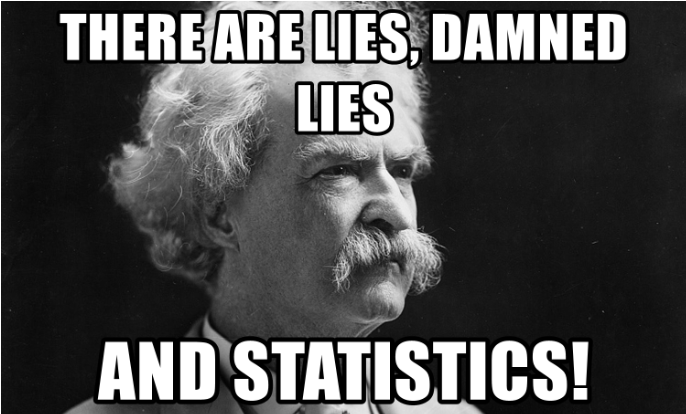This is just a joke which seems to suggest that any measure of central tendency can give a wrong idea about a collection of objects. Read on to understand what that fraction of a human actually means in the language of mathematics.

## The Big Idea: Measures of Central Tendency

### A simple idea: Know about a group better

When the tallest child in the class is 6'01", and the shortest one is just 4'9", but, these two numbers don't tell you too much about the class composition with regards to the height of the students. However, if you were told that the mean height of the class is 5'6", it would give you a rough idea of how tall everyone is in general. Now if I told you that in Standard 7, the average heights of sections A, B, and C respectively are 5'1", 5'6", and 5'9", you could conclude that the children of Section C are taller than the children of Section A. This is one example that tells us how essential measures of central tendency are. The idea is to understand the different measures of central tendency and how to calculate them. The three measures of central tendency are mean, median and mode. The mean is also referred to commonly as average.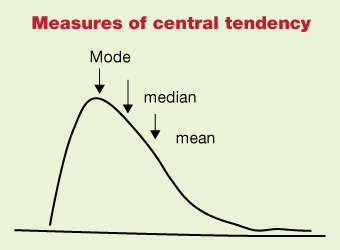To understand the definition of these three, let us look at the weights of 10 boys in kilograms: 45, 39, 53, 45, 43, 48, 50, 40, 40, 45.

### Mean or Average

This is the most common central tendency we know about and use. It is also known as average. It is simply the sum of all the components in a group or collection, divided by the number of components. So, in the above example, there are 10 components.

\begin{align} {\text{Therefore the average of the group}}& = \frac{{\left( {45 + 39 + 53 + 45 + 43 + 48 + 50 + 40 + 40 + 45} \right)}}{{10}}\\ & = \frac{{448}}{{10}}\\ & = 44.8 \end{align}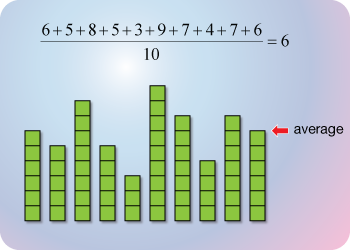So, the average (or mean) weight of those 10 boys is 44.8 kgs. Let us see how it looks when we arrange the weights in ascending order: 39, 40, 40, 43, 45, 45, 45, 48, 50, 53. We see that 4 boys have a weight less than the mean of the group, while 6 boys have weights higher than the mean of the group.

### Median

For finding this measure of central tendency, it is necessary to write the components of the group in increasing order.

\begin{align} &{\text{So, 45, 39, 53, 45, 43, 48, 50, 40, 40, 45}} \\ &{\text{needs to be written as}} \\ &{\text{39, 40, 40, 43, 45, 45, 45, 48, 50, 53}} \end{align}

The median is the middle value of a set of numbers arranged in increasing order. So, if we need to find the median height of a set of 5 children whose heights we know, then we need to arrange the 5 heights in ascending order, and the third height is the median. But what happens if the collection of numbers has even number of components. In such cases, we take the average (mean) of the two middlemost numbers. So, let us go back to our collection of 10 numbers:

\begin{align} &{\text{45, 39, 53, 45, 43, 48, 50, 40, 40, 45}} \end{align}

We have already rearranged the numbers in ascending order as

\begin{align} &{\text{39, 40, 40, 43, 45, 45, 45, 48, 50, 53}} \end{align}

The two middlemost numbers are now marked in red here:

\begin{align} &{\text{39, 40, 40, 43,}} \end{align}\begin{align} &{\text{45, 45,}} \end{align}\begin{align} &{\text{45, 48, 50, 53}} \end{align}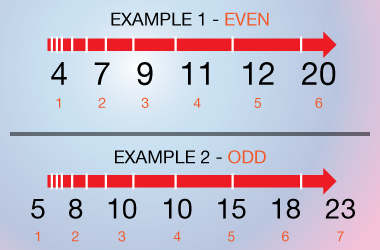## The mean of these two numbers is 45, so the median is also 45.

### Mode

In any collection of numbers, the number which occurs the most number of times is the mode. Let us continue with this same collection of numbers 39, 40, 40, 43, 45, 45, 45, 48, 50, 53. Let us try to mark in different colors those numbers which occur multiple times.

39, 40, 40, 43, 45, 45, 45, 48, 50, 53. So 40 occurs twice, and 45 occurs thrice, and all the other 5 numbers occur once each. So, for this collection, 45 is the mode. As you can see, it is not necessary to arrange the collection of numbers in ascending order to find the mode.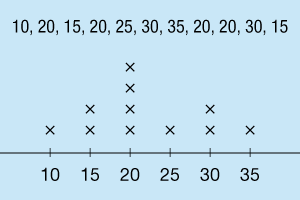More Important Topics
Numbers
Algebra
Geometry
Measurement
Money
Data
Trigonometry
Calculus
More Important Topics
Numbers
Algebra
Geometry
Measurement
Money
Data
Trigonometry
Calculus
Learn from the best math teachers and top your exams

• Live one on one classroom and doubt clearing
• Practice worksheets in and after class for conceptual clarity
• Personalized curriculum to keep up with school

0